## Formatting on the Tricki

You can experiment with markup and other features of the Tricki in the Sandbox.

What it looks like What you type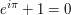(Euler's identity)
[maths euler|Euler's identity] e^{i \pi} + 1 = 0[/maths]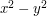can be factorised as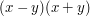. $x^2 - y^2$ can be factorised as $(x-y)(x+y)$.
Some shortcuts: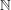,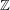,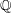,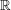,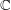. Some shortcuts: $\N$, $\Z$, $\Q$, $\R$, $\C$.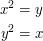[maths]\begin{align} x^2 &= y \\ y^2 &= x \end{align}[/maths]
Link to Tricki article How to use Zorn's lemma. [[How to use Zorn's lemma]]
Link to the same article. [[How to use Zorn's lemma|same article]]
Blending: we do not have many Dummy articles. [[Dummy article]]s
Link to a non-article page on the Tricki. Link to a [[forum/|non-article page on the Tricki]].
Link to Wikipedia article on mathematics. Link to [[w:Mathematics|Wikipedia article on mathematics]].
Link to preprint on the arXiv. Link to [[arxiv:math/0408230|preprint on the arXiv]].
Link to review on MathSciNet. Link to [[mathscinet:16335|review on MathSciNet]].
Link to Google search. Link to [[google:Mathematics|Google search]].
External link to the Prime Pages. External link to [http://primes.utm.edu/ the Prime Pages].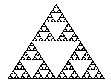[image sierpinski.gif|Sierpinski triangle]Sierpinski triangle
Some text around the image. It will wrap around to the right of the image.
[image sierpinski.gif|left|Sierpinski triangle]
Reference to (Euler's identity) above. Reference to [eqref euler] above.
Theorem 1 (Fundamental theorem of basic arithmetic) Let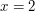. Then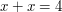.
[theorem ftba|Fundamental theorem of basic arithmetic]Let $x = 2$. Then $x + x = 4$.[/theorem]
Note: always use descriptive IDs and not position dependent ones in order to make restructuring articles earier.
Proof of Theorem 1. This is obvious.
[proof ftba]This is obvious.[/proof]
By Theorem 1,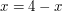. By [ref Theorem #ftba], $x = 4 - x$.

### Example 1

This is an example section; see the formatting sections page for more information.
[EXAMPLE extag] This is an example section; see the [[tricki/help/formatting-structure|formatting sections]] page for more information.[/EXAMPLE]
Here is a reference to Example 1. Here is a [ref reference to Example #extag].
You can make bits of text bold, italic or even both. You can make bits of text '''bold''', ''italic'' or '''''even both'''''.
• You can
• also
• create
• lists!
 * You can * also ** create ** lists! 
1. You can
2. also
1. create
2. numbered lists!
 # You can # also ## create ## numbered lists! 

 === Heading 1 === ==== Heading 2 ==== 
Link to heading 1 above. [[#Heading 1|Link to heading 1]] above.
Some hidden text here. (You've found the hidden text!) [cut]Some hidden text here.||You've found the hidden text![/cut]
The proof is obvious. Indeed, 2 + 2 = 4. [add]The proof is obvious.{{ Indeed, 2 + 2 = 4.}}[/add]
Lemma 2 (The Important Lemma)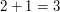[frame][lemma important|The Important Lemma] $2 + 1 = 3$ [/lemma][/frame]
Yőü can ûse TeX-stylè acceñts. Y\H{o}\"u can \^use TeX-styl\e acce\~nts.
You can also use TeX-style dashes — Birch–Swinnerton-Dyer conjecture. You can also use TeX-style dashes --- Birch--Swinnerton-Dyer conjecture.
 Cell (1,1) Cell (1,2) Cell (2,1) Cell (2,2)
<table border="1"> <tr><td>Cell (1,1)</td><td>Cell (1,2)</td></tr> <tr><td>Cell (2,1)</td><td>Cell (2,2)</td></tr> </table>
See, for example, the W3Schools page on tables for more information and examples.
Editorial notes: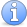Incomplete This article is incomplete. This article is missing large parts of what is required of a Tricki article.
[note article incomplete]This article is missing large parts of what is required of a Tricki article. [/note]
Notes can be specified at the article level and the section level. They can be one of three types: 'incomplete', 'contributions wanted', or 'attention'. See the page about editorial markup for more information.

Available theorem-like environments:

• theorem (numbering: theorem)

• lemma (numbering: theorem)

• corollary (numbering: theorem)

• conjecture (numbering: theorem)

• proposition (numbering: theorem)

• sublemma (numbering: theorem)

• problem (numbering: problem)

• exercise (numbering: problem)

• question (numbering: problem)

• definition (numbering: definition)

• claim (no numbering)

• remark (no numbering)

• remarks (no numbering)

The theorem-type environments are rendered in italics; the others are not.

Lists can be nested to an arbitrary depth by putting more asterisks at the beginning of the line.

There are three levels of headings (from === to =====).

Images can also be floated to the right (right instead of left) or centred (centre`).